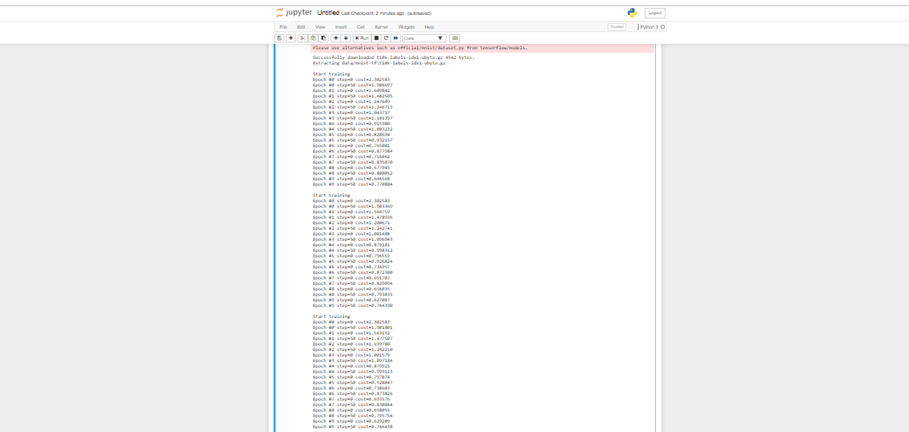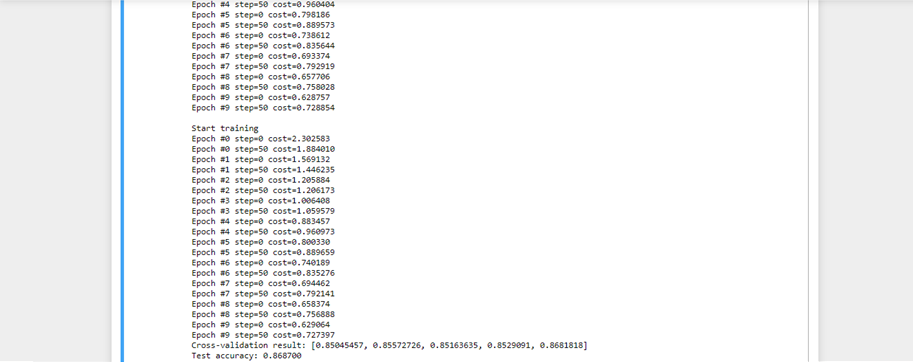# Does TensorFlow have cross validation implemented for its users?

1 view

I was thinking of trying to choose hyperparameters (like regularization for example) using cross-validation or maybe train multiple initializations of models and then choose the model with highest cross-validation accuracy. Implementing k-fold or CV is simple but tedious/annoying (especially if I am trying to train different models in different CPU's, GPU's or even different computers, etc). I would expect a library like TensorFlow to have something like this implemented for its user so that we don't have to code the same thing 100 times. Thus, does TensorFlow have a library or something that can help me do Cross Validation?

As an update, it seems one could use scikit learn or something else to do this. If this is the case, then if anyone can provide a simple example of NN training and cross-validation with scikit learn it would be awesome! Not sure if this scales to multiple CPUs, GPUs, clusters, etc though.

by (8k points)

As already discussed, tensorflow doesn't provide its own way to cross-validate the model. The recommended way is to use KFold. It's a bit tedious, but doable. Here's a complete example of cross-validating MNIST model with tensorflow and KFold:

from sklearn.model_selection import KFold

import tensorflow as tf

from tensorflow.examples.tutorials.mnist import input_data

# Parameters

learning_rate = 0.01

batch_size = 500

# TF graph

x = tf.placeholder(tf.float32, [None, 784])

y = tf.placeholder(tf.float32, [None, 10])

W = tf.Variable(tf.zeros([784, 10]))

b = tf.Variable(tf.zeros())

pred = tf.nn.softmax(tf.matmul(x, W) + b)

cost = tf.reduce_mean(-tf.reduce_sum(y*tf.log(pred), reduction_indices=1))

correct_prediction = tf.equal(tf.argmax(pred, 1), tf.argmax(y, 1))

accuracy = tf.reduce_mean(tf.cast(correct_prediction, tf.float32))

init = tf.global_variables_initializer()

train_x_all = mnist.train.images

train_y_all = mnist.train.labels

test_x = mnist.test.images

test_y = mnist.test.labels

def run_train(session, train_x, train_y):

print "\nStart training"

session.run(init)

for epoch in range(10):

total_batch = int(train_x.shape / batch_size)

for i in range(total_batch):

batch_x = train_x[i*batch_size:(i+1)*batch_size]

batch_y = train_y[i*batch_size:(i+1)*batch_size]

_, c = session.run([optimizer, cost], feed_dict={x: batch_x, y: batch_y})

if i % 50 == 0:

print "Epoch #%d step=%d cost=%f" % (epoch, i, c)

def cross_validate(session, split_size=5):

results = []

kf = KFold(n_splits=split_size)

for train_idx, val_idx in kf.split(train_x_all, train_y_all):

train_x = train_x_all[train_idx]

train_y = train_y_all[train_idx]

val_x = train_x_all[val_idx]

val_y = train_y_all[val_idx]

run_train(session, train_x, train_y)

results.append(session.run(accuracy, feed_dict={x: val_x, y: val_y}))

return results

with tf.Session() as session:

result = cross_validate(session)

print "Cross-validation result: %s" % result

print "Test accuracy: %f" % session.run(accuracy, feed_dict={x: test_x, y: test_y})